# How to build a Machine Learning Intrusion Detection system

## Introduction

Machine learning techniques are changing our view of the world and they are impacting all aspects of our daily life. Thus machine learning is playing a huge role in information security. In this module you will not only explore the fundamentals behind machine learning techniques but you will dive into a hands-on experience to learn how to build real world Intrusion detection systems from scratch using cutting edge techniques, programming libraries and publicly available datasets.

This module will cover:

• Machine learning models
• The required steps to build a Machine learning project
• How to evaluate a machine learning Model
• Most useful Data Science and Machine learning libraries
• Artificial Neural Networks and Deep Learning
• Next Generation Intrusion detection systems using Machine learning Techniques.

## Artificial intelligence

Artificial intelligence is the art of making computer programs to behave like a human and by behave i mean perceiving, learning, understanding and knowing. AI is involving many areas such as computer science, neuroscience, psychology and so on.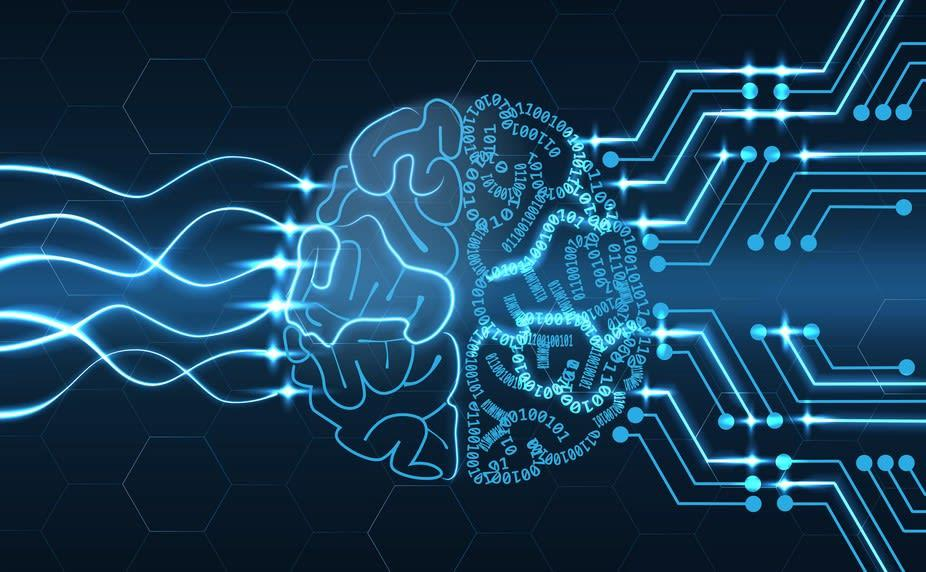## Machine Learning models

Machine learning is the study and the creation of algorithms that learn from given data and examples. It is a particular approach to artificial intelligence.Tom M. Mitchell (an american computer scientist ) defines machine learning as "A computer program is said to learn from experience E with respect to some class of tasks T and performance measure P if its performance at tasks in T, as measured by P, improves with experience E" . In machine learning we have four major models; supervised, semi-supervised,unsupervised and reinforcement.

I. Supervised learning: if we have the Input and the Output variable then it is a supervised learning. In this case we only need to map the function between the inputs and the outputs. Supervised learning could be divided into two other sub-categories; Classification and regression: - Classification: when the output is a categorical variable - Regression: when the output variables are continuous values.

Let's discover some supervised learning algorithms:

• Naive Bayes: this classification algorithm is based on the the Bayes' theorem.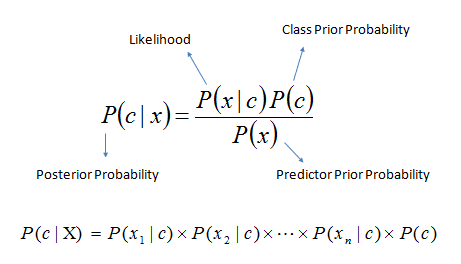• Decision Trees: are machine learning algorithms that predict the possible outputs thanks to a tree-like graph,the entire data is represented as a root node and the final leafs are called Terminal Nodes.Dividable nodes are known as Decision Nodes.
• Support Vector Machines: are binary classifiers used to identify a separating hyper-plane of data that are represented in a multi-dimensional space.Thus, that hyper-plane is not necessary a simple line.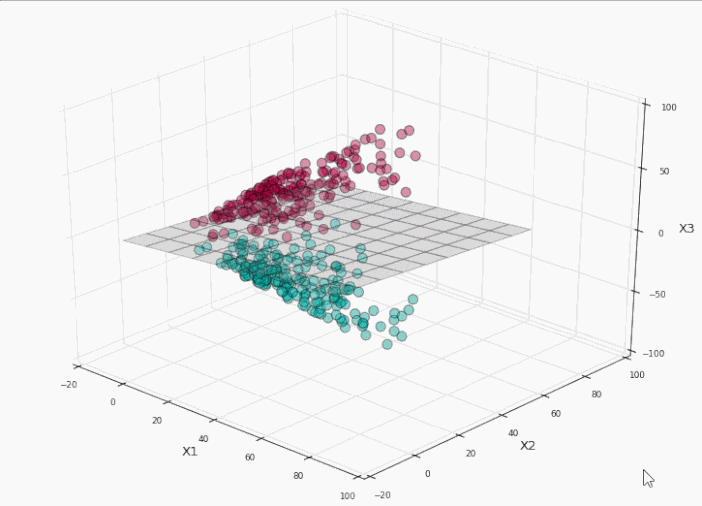II. Semi-supervised: this model is not fully supervised while it contains both labeled and unlabeled data. This model is used generally to improve the learning accuracy. - Unsupervised: If we don't have information about the output variables then it is unsupervised learning.The model is trained totally with unlabeled data.Clustering is one of the most well known unsupervised techniques.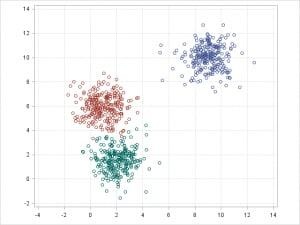III. Reinforcement: in this model the agent is being optimized based on the feedback from the environment (the reward)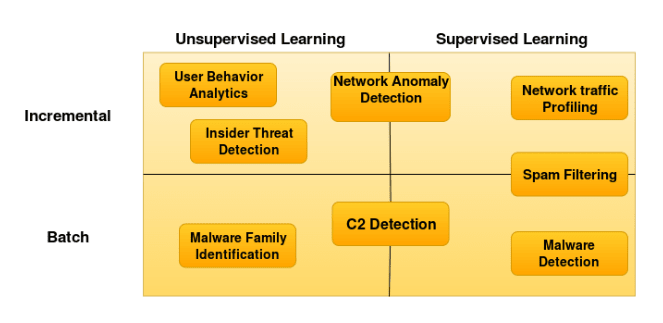## Machine learning steps

In order to build a Machine learning model our project need to follow two major phases; training and experimenting. During the training phase a feature engineering operation is needed because it is critical to feed the machine learning model with a well defined features. Not all the data is useful in our project. After choosing the machine learning algorithm that we are going to use, we feed it by the chosen data. After training, we need to put the model into a test or what we call an experience to evaluate the model based on many evaluation metrics.

### Machine learning evaluation metrics

Building a machine learning model is a methodological process. Thus, in order to test our machine learning model performance we need to use a well-defined metrics based on scientific formulas: all these formulas are needing four parameters; false positive, true positive, false negative and true negative.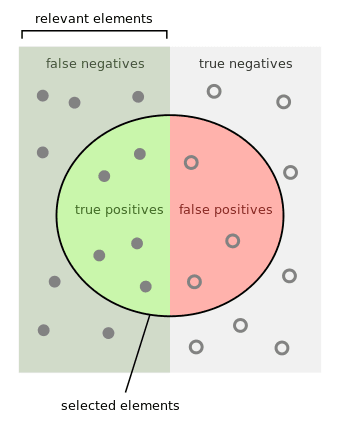Notation

• tp = True Positive
• fp = False Positive
• tn = True Negative
• fn = False Negative

#### Precision

Precision or Positive Predictive Value, is the ratio of the positive samples that are correctly classified by the the total number of positive classified samples.Simply it is the number of the found samples were correct hits.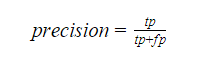#### Recall

Recall or True Positive Rate, is the ratio of true positive classifications by the total number of positive samples in the dataset. It represents how many of the true positives were found.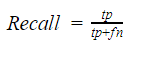#### F-Score

F-Score of F-Measure, is a measure that combines precision and recall in a one harmonic formula

#### Accuracy

Accuracy is the ratio of the total correctly classified samples by the total number of samples. This measure is not sufficient by itself,because it is used when we have equal number of classes.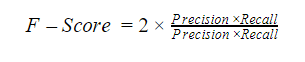#### Confusion Matrix

Confusion matrix is is a table that is often used to describe the performance of a classification model.

### Machine learning python frameworks

As a programming language we used python for many reasons. First comparing to other languages it is more productive and flexible than Java and C++.According to thestateofai.com 78% of developers are using python in their Artificial intelligence projects that means a better documentation and support from the development community. Python is coming with external, easy and advanced machine learning packages in terms of run-time and complexity. The following are some of the most used Python libraries in Machine learning:

SciPy : it is used for mathematics and engineering field in general

NumPy : it is used to manipulate large multi-dimensional arrays and linear algebra

MatplotLib : it provides great data visualization capabilities including: Confusion Matrix,Hitmaps,linear plots

Tensorflow : is an open-source library for machine intelligence and numerical computation developed by Google Brain Team within Google's Machine Intelligence research organization .You can deploy computation to one or more CPUs and GPUs.

Keras : is an open-source neural network library written in Python running on top of TensorFlow to ease the experimentation and the evaluation of the neural networks model.

Theano : is an open source neural network library written in Python running on top of TensorFlow to ease the experimentation and the evaluation of the neural networks model.

To install any Python library this command will do the job : pip install Package-Here

The following graph illustrates a comparison between some machine learning frameworks made by Favio Vázquez especially Deep learning frameworks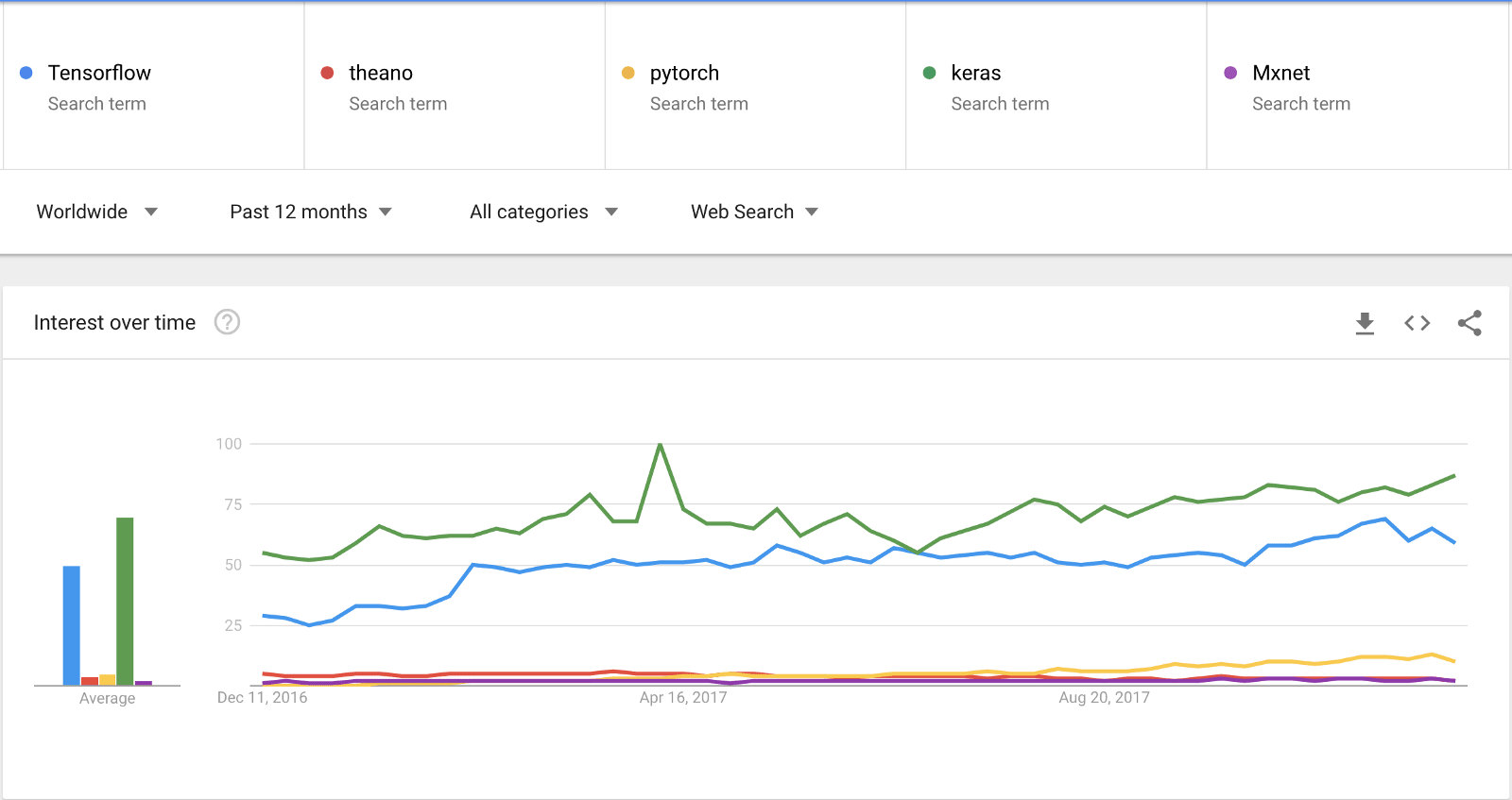Wait, but what is Deep Learning?

### Artificial Neural networks and Deep Learning:

The main goal of Artificial neural networks is to mimic how the brain works.To have a better understanding let's explore how a human brain actually works.Human brain is a fascinated complex entity with many different regions to perform various tasks like listening, seeing, tasting and so on. If the human brain is using many regions to perform multiple tasks so logically every region act using a specific algorithm for example an algorithm for seeing, an algorithm for hearing etc...Right? Wrong! The brain is working using ONE Algorithm. This hypothesis It is called The "one learning algorithm" hypothesis. There is some evidence that the human brain uses essentially the same algorithm to understand many different input modalities. For more information check Ferret experiments, in which the "input" for vision was plugged into auditory part of brain, and the auditory cortex learns to "see." The cell that compose the neuron system is called a neuron.The information transmission is happening using electrochemical signalling and propagation is done thanks to the neuron dendrites.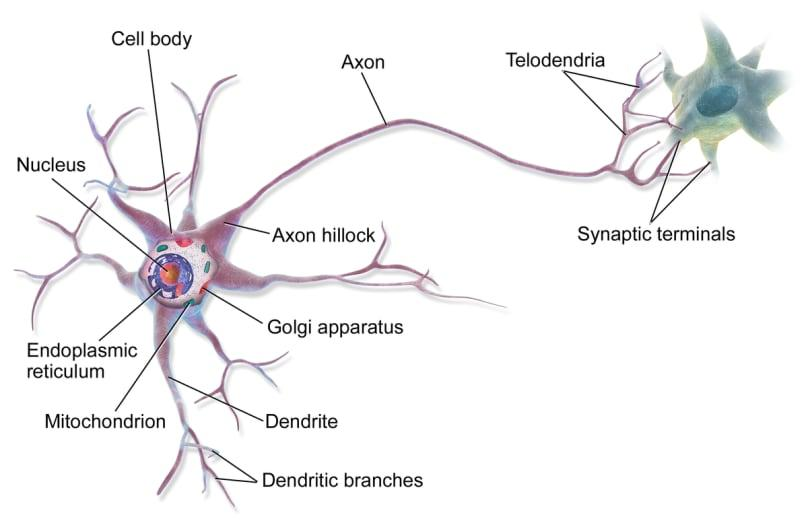The analogy of the human brain neuron in machine learning is called a perceptron. All the input data is summed and the output applies an activation function. We can see activation functions as information gates.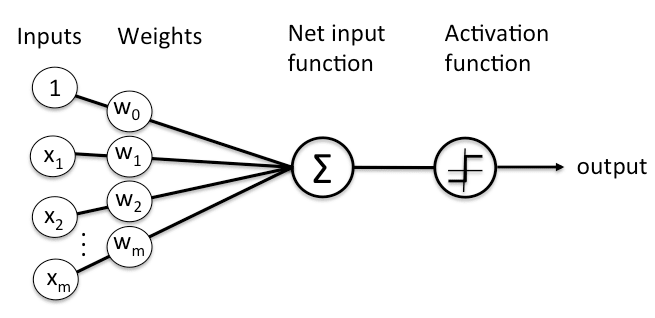PS: " The analogy between a perceptron and a human neuron is not totally correct. It is used just to give a glimpse about how a perceptron works. The human mind is so far more complicated than Artificial neural networks. There are few similarities but a comparison between the mind and Neural networks is not really correct."

There are many used activation functions:

• Step Function : Every output node have a predefined threshold value
• Sigmoid Function : Sigmoid functions are one of the most widely used activation functions
• Tanh Function : Another activation function used is the Tanh function
• ReLu Function : It is also called a rectified linear unit.It gives an output x if x is positive and 0 otherwise.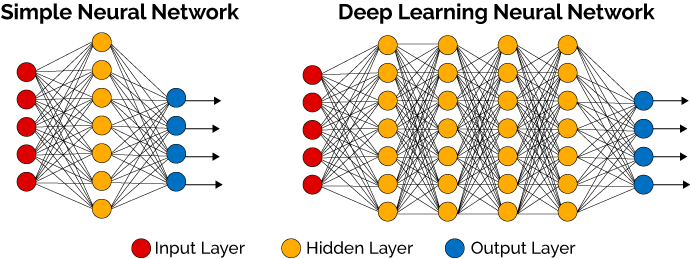Many connected perceptrons build a simple neural network that consists of three parts: Input layer,hidden layer and an output layer.The hidden layer is playing the inter-communication role in the neural network or sometimes what what we call a Multi-layer perceptron network. If we have more than 3 hidden layers then we are talking about Deep Learning and Deep learning Networks.According to the data scientist and deep learning experts like the machine learning practitioner Dr. Jason Brownlee; every deep learning model must go thru five steps:

Network Definition: in this phase we need to define the layers.Thanks to Keras this step is easy because it defines neural networks as sequences and to define layers we just need to create a sequence instance with mentioning the number of outputs

Network Compiling: Now we need to compile the network including choosing the optimizing technique like Stochastic Gradient Descent (sgd) and a Loss function (Loss function is used to measure the degree of fit) to evaluate the model we can use Mean Squared Error (mse)

Network Fitting: a Back-Propagation algorithm is used during this step based on the parameters specified in the compiling step.

Network Evaluation : After fitting the network an evaluation operation is needed to evaluate the performance of the model

Prediction: Finally after training the deep learningmodel we now can use it to predict a new malware sample using a testingdataset

## Intrusion detection systems with Machine learning

Dangerous hackers are inventing new techniques in a daily basis to bypass security layers and avoid detection.Thus it is time to figure out new techniques to defend against cyber threats. Intrusion detection systems are a set of devices or pieces of software that play a huge role in modern organizations to defend against intrusions and malicious activities.We have two major intrusion detection system categories:

• Host Based Intrusion Detection Systems (HIDS): they run on the enterprise hosts to
• Network Based Intrusion Detection Systems (NIDS): their role is to detect network anomalies by monitoring the inbound and outbound traffic.

The detection can be done using two intrusion detection techniques:

• Signature based detection technique: the traffic is compared against a database of signatures of known threats
• Anomaly-based intrusion technique: inspects the traffic based on the behavior of activities.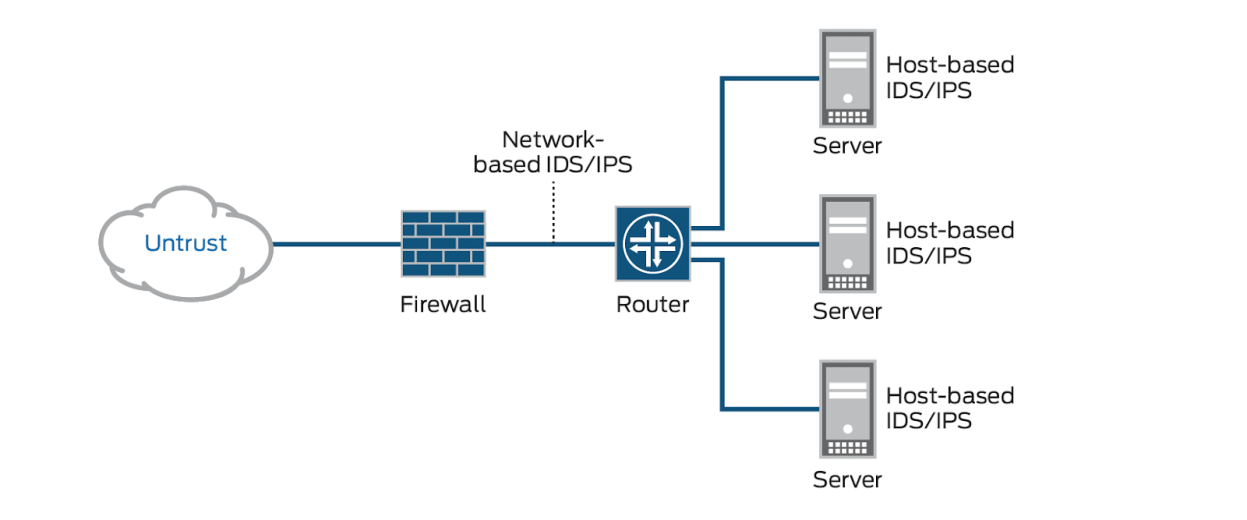Modern organization are facing thousands of threats in a daily basis.That is way the classic techniques could not be a wise solution to defend against them.Many researchers and information security professionals are coming with new concepts,prototypes or models to try solving this serious security issues.For example this is graph shows the different intrusion detection techniques including the discussed machine learning algorithms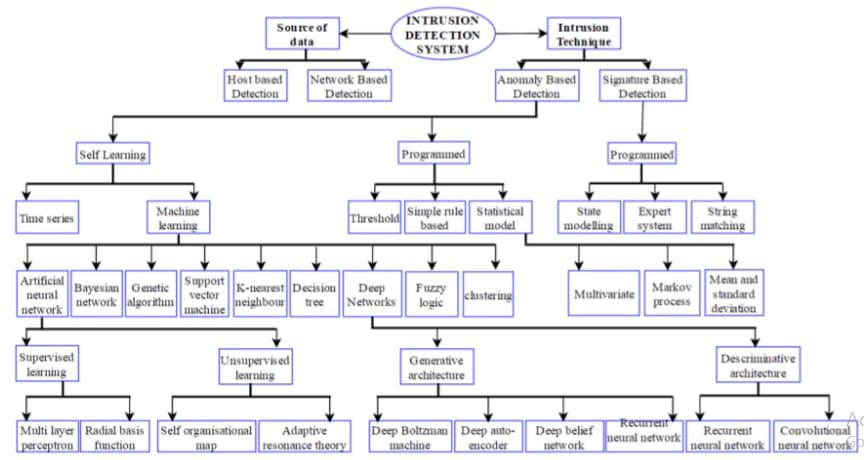By now, after reading the previous sections we are able to build a Machine learning detection system. As discussed before the first step is Data processing.The are many publicly available datasets in the wild used by data scientist to train machine learning models.You can download some of them from here:

The NSL-KDD is one of the most used datasets in intrusion detection anomaly based models.It contains different attacks categories: DoS, Probe, U2R and R2L.

It is an enhanced dataset from the KDD99 dataset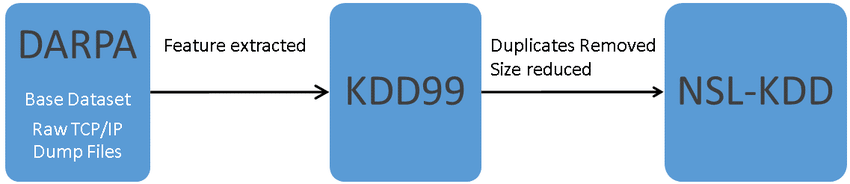After choosing the feature that you are going to work on and splitting the dataset into two sub-datasets for the training and the experience (They should not be the same) you can choose one of the machine learning algorithms represented in the graph of intrusion detection techniques and train your model.Finally when you finish the training phase it is time to put your model to the test and check its accuracy based on the machine learning evaluation metrics. To explore some of the tested models i recommend taking an eye on "Shallow and Deep Networks Intrusion Detection System: A Taxonomy and Survey" research paper.

There are a lot of talks about the promise of machine learning or AI ininformation security but in the other side there is a debate and some concerns about it. To discover more about Machine learning promises in cyber security it is highly recommended to watch Thomas Dullien Talk : " Machine Learning, offense, and the future of automation" from here:

You can also download the presentation slides from this link: Presentation Slides

## Summary

This article is a fair overview of machine learning in information security.We discussed the required fundamentals in every machine learning project starting from the fundamentals to gaining the skills to build a machine learning projects.We took intrusion detection systems as real world case study.